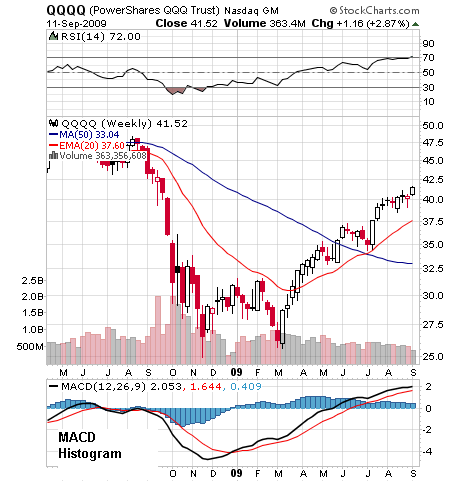The moving average convergence divergence indicator (MACD) is a trend following indicator showing when a trend is gaining strength or losing momentum as it moves above or below a central zero line. Here is an example:-Chart supplied by stockcharts.com. Your broker will supply charting software.

The MACD line is calculated for you and this is what it is made up from:-

1. A 12 period EMA is calculated.

2. A 26 period EMA is calculated.

3. The 26 period EMA is subtracted from the 12 period EMA to create the MACD line (the darker line in the histogram.)

Also shown on the histogram are:-

4. The MACD line is used to calculate a 9 period EMA to give the signal line (the lighter line in the histogram.)

What does it mean?